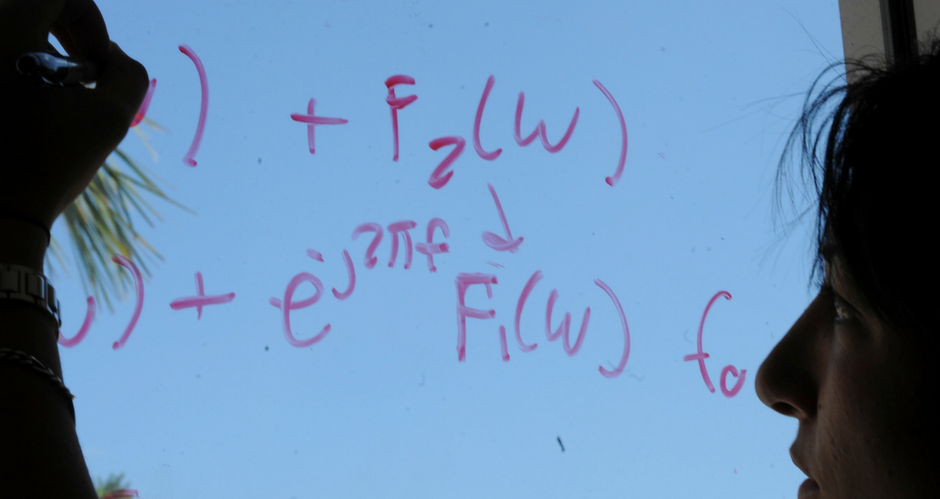## Mathematics and System Engineering Faculty Publications

### A sturm separation theorem for a linear 2nth order self-adjoint differential equation

Article

#### Publication Title

Journal of Applied Mathematics and Stochastic Analysis

#### Abstract

For the 2nth order equation, (−1)nv(2n)+qv=0, with q continuous, we obtain a Sturm Separation theorem, involving n+1 solutions of the equation, which is somewhat analogous to the classical result that the zeros of two linearly independent solutions of the second order equation separate each other.

29

46

#### DOI

10.1155/S1048953395000037

1995

COinS